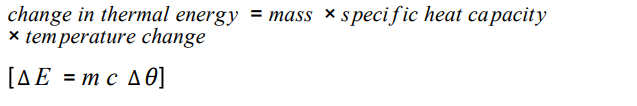# AQA GCSE Physics Temperature changes in a system and specific heat capacity Focus Questions

1. If a system is heated its temperature will increase. What three things does the increase in temperature depend on?
2. Look at this equation.3. What is the unit of mass?
4. What is the unit of change in thermal energy?
5. What is the unit of specific heat capacity?
6. What is the unit of temperature change?
7. What does the triangle mean in the equation?
8. Rearrange so that m = and c =
9. Write down the definition of specific heat capacity.